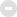#### 热帖## 『健康时刻』 <!-- var health_message_7ree=""; day = new Date( ); hr = day.getHours( ); if (( hr >= 0 ) && (hr <= 1 )) health_message_7ree="0点~1点：进入睡眠状态，充分恢复体能。" if (( hr >= 1 ) && (hr <= 2 )) health_message_7ree="1点~2点：人体进入浅睡眠阶段，易醒，对痛觉特别敏感。" if (( hr >= 2 ) && (hr <= 3 )) health_message_7ree=" 体内大部分器官处于一天中工作最慢的时刻。肝脏紧张地工作，为人体排毒。" if (( hr >= 3 ) && (hr <= 4 )) health_message_7ree="3点~4点：全身处于休息状态，肌肉完全放松。" if (( hr >= 4 ) && (hr <= 5 )) health_message_7ree="4点~5点：血压最低，人体脑部供血最少。所以，此时老年人容易发生心脑血管意外。" if (( hr >= 5 ) && (hr <= 6 )) health_message_7ree="5点~6点：经历了一定时间的睡眠，人体得到了充分休息。此时起床，显得精神饱满。" if (( hr >= 6 ) && (hr <= 7 )) health_message_7ree="6点~7点：血压开始升高，心跳也逐渐加快。" if (( hr >= 7 ) && (hr <= 8 )) health_message_7ree="7点~8点：体温开始上升，人体免疫力最强。" if (( hr >= 8 ) && (hr <= 9 )) health_message_7ree="8点~9点：皮肤有毒物质排除殆尽，性激素含量最高。" if (( hr >= 9 ) && (hr <= 10 )) health_message_7ree="9点~10点：皮肤痛觉降低。此时是就医注射的好时机。" if (( hr >= 10 ) && (hr <= 11 )) health_message_7ree="10点~11点：精力充沛，最适宜工作。" if (( hr >= 11 ) && (hr <= 12 )) health_message_7ree="11点~12点：精力最旺盛，人体不易感觉疲劳。" if (( hr >= 12 ) && (hr <= 13 )) health_message_7ree="12点~13点：经历了一个上午的工作，人体需要休息。" if (( hr >= 13 ) && (hr <= 14 )) health_message_7ree="13点~14点：胃液分泌最多，胃肠加紧工作，适宜进餐，稍感疲乏，需要短时间的休息。" if (( hr >= 14 ) && (hr <= 15 )) health_message_7ree="14点~15点：人体应激能力下降，全身反应迟钝。" if (( hr >= 15 ) && (hr <= 16 )) health_message_7ree="15点~16点：体温最高，工作能力开始恢复。" if (( hr >= 16 ) && (hr <= 17 )) health_message_7ree="16点~17点：血糖升高，脸部最红。" if (( hr >= 17 ) && (hr <= 18 )) health_message_7ree="17点~18点：工作效率最高，肺部呼吸运动最活跃，适宜进行体育锻炼。" if (( hr >= 18 ) && (hr <= 19 )) health_message_7ree="18点~19点：人体痛觉再度降低。" if (( hr >= 19 ) && (hr <= 20 )) health_message_7ree="19点~20点：血压略有升高。此时，人们情绪最不稳定。" if (( hr >= 20 ) && (hr <= 21 )) health_message_7ree="20点~21点：记忆力最强，大脑反应异常迅速。" if (( hr >= 21 ) && (hr <= 22 )) health_message_7ree="21点~22点：脑神经反应活跃，适宜学习和记忆。" if (( hr >= 22 ) && (hr <= 23 )) health_message_7ree="22点~23点：呼吸开始减慢，体温逐渐下降。" if (( hr >= 23 ) && (hr <= 24 )) health_message_7ree="23点~24点：机体功能处于休息状态，一天的疲劳开始缓解。" document.write(health_message_7ree) //--->分区版主: 小羊, glzjin, Coxxs

## 站务专版## 社区公告

42 / 2759263 / 2126